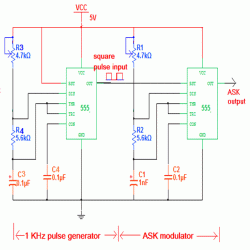## Thursday, February 19, 2015

### Circuit ASK Modulator Diagram using 555 Timer IC

Robotronicdiagram. - Here is Circuit ASK Modulator Diagram using 555 Timer IC. ASK means Amplitude Shift Keying (also known as ON-OFF keying). It’s one of the Modulation Scheme used to transmit Digital Data using High Frequency carrier signal. It’s very simple and popular method. The circuit diagram like in figure 1 below.

Circuit DiagramFigure 1. Circuit ASK Modulator Diagram using 555 Timer IC(Source: www.engineersgarage.com)

Description

Circuit diagram like in figure 1 above is Circuit ASK Modulator Diagram using 555 Timer IC. The first Waveform is bit pattern – data bits. 2nd Waveform shows ASK Modulation – for data bit 1 carrier signal (with frequency Fc) present but for data bit 0 there is no carrier signal. Usually carrier frequencies are higher than (100s of KHz) data rate. So in Single bit period several cycles of carrier are transmitted.

Here given Circuit demonstrates how ASK Modulated wave can be generated. The Circuit is build using IC555. The bit stream can be given as input, and as an output IC555 generates ASK Wave. In this experiment instead of bit-stream, Square pulses are applied as input that can represent Logic High and Logic Low as bit 1 and bit 0. To generate Square pulses one more IC555 is used.

Circuit Description

There are two sections in the circuit

2.      1 KHz Pulse Generator

Pulse Generator

IC555 is connected in Astable mode. It will generate continuous pulses at 1 KHz frequency. The frequency is determined by RC components R4, R5 and C3 as

F = 1.44 / (R4 + 2×R5) × C3

This 1 KHz pulse output is connected to reset pin (4) input of 2nd IC555 that works as ASK Modulator.

As shown in figure IC 555 is configured as Astable Multi vibrator. When its reset pin is high it generates continuous Square pulses with frequency

F = 1.44 / (R1 +2×R2) × C1

And when its reset pin is low its output is also low. The values of R1, R2 and C1 are selected as to get desire carrier frequency. The carrier frequency is selected as 100 KHz. Let us see how values of R1, R2 and C1 are calculated.

Thank you for your coming here in www.robotronicdiagram.com, we hope the article above will help you to know more about your information in electronic circuit design and other in this time. Please comment here when you want to share and other. Thank you.